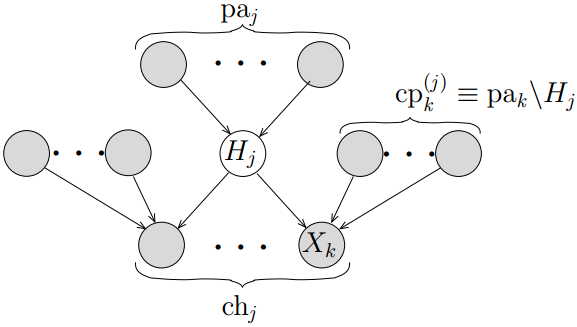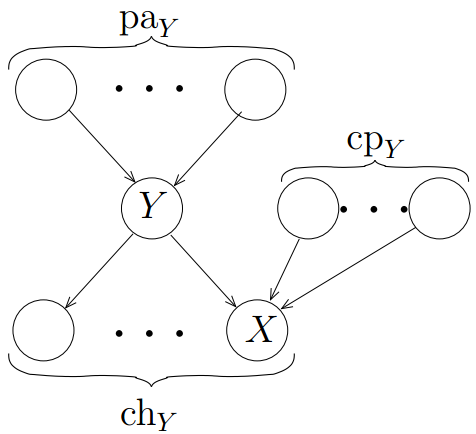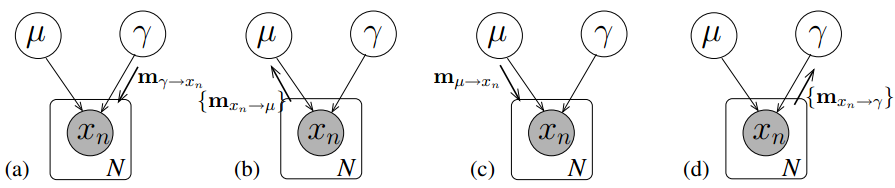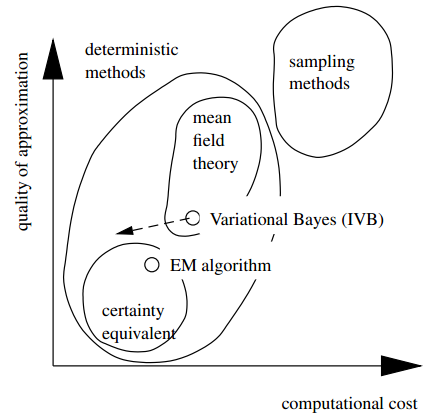# 变分消息传播模型与算法

## Variational Message Passing

Posted by huajh7 on March 23, 2013

### 理论基础

#### Bayesian networks#### Exponential Family

$(X,B\|{p_\theta }:\theta \in \Theta \|)$是可控参数统计结构，其密度函数可表示为如下形式：

#### Sufficient Statistics

$X$积分有，

### 变分消息传播模型

#### 变分分布Q在共轭指数模型下的最优化$\phi_{XY}(X,\beta ) = \left[ \begin{array}{l}\beta X\\ - \beta /2\end{array} \right]$可以重参数化为${\tilde{\phi} _{XY}}(\langle u_X \rangle ,\langle {u_\beta} \rangle ) = \left[ \begin{array}{l} \langle {u_\beta} \rangle_{0} \langle u_X \rangle_{0} \\ - \langle {u_\beta} \rangle_{0} /2 \end{array} \right]$

#### 变分消息传播模型

$L\left( Q \right)$被分解为每一个节点上的贡献值 $\left\{ L_i \right\}$，如节点${H_j}$的贡献值为

### 变分消息传播算法

#### 变分消息的定义

$\begin{equation} m_{Y \to X} = \left\langle {u_Y}\right\rangle. \end{equation} \tag{3}$

$\phi_Y^* = \tilde{\phi_Y}\left( \left\{ m_{i \to Y} \right\}_{i \in p{a_Y}} \right) + \sum\limits_{j \in c{h_Y}} m_{j \to Y} \tag{5}$.

#### 算法描述

 Step1. 通过初始化相关的矩向量$\left\langle {u_j({X_j})} \right\rangle$来初始化每个因子分布${Q_j}$.
 Step2. 对于每一个节点${X_j}$，
 1) 从父节点和子节点接收 (3),(4) 式所定义的消息。 前提是子节点已经从${X_j}$的co-parents接收到消息。 2) 使用 (5) 式更新自然参数向量$\phi _j^*$； 3) 根据新的参数向量更新距向量$\left\langle {u_j({X_j})} \right\rangle$；
 Step3. 计算新的下界$L(Q)$;
 Step4. 如果经过数次迭代已经无法增加下界值，或者各边缘分布达到稳定值，则结束；否则回到Step2。### 算法分析

#### VB算法与EM算法比较

EM算法计算随机变量 (或归类于参数) 后验分布的点估计，但估计隐变量的真实后验分布。用这些参数的众数作为点估计，无任何其他信息。 而在VB算法作为一个分布估计 (Distributional Approximation) 方法，计算所有变量的真实后验分布的估计，包括参数和隐变量。 在贝叶斯推断中，计算点估计一般使用常用的均值而非众数。与此同时，应该注意的是计算参数在VB中与EM有不同的意义。 EM算法计算贝叶斯网络本身的参数的最优值。而VB计算用于近似参数和隐变量的贝叶斯网络的参数最佳值，VB会先找一个合适的参数分布， 通常是一个先验分布的形式，然后计算这个分布的参数值，更准确说是超参数，最后得到联合分布的各参数的分布。

#### 算法复杂性### 参考文献

 John M. Winn, M. Bishop, Variational Message Passing, Journal of Machine Learning Research, 2004

 John M. Winn, Variational Message Passing and its Applications, University of Cambridge, 2003

 Michael I. Jordan, An Introduction to Variational Methods for Graphical Models, Machine Learning, 1999